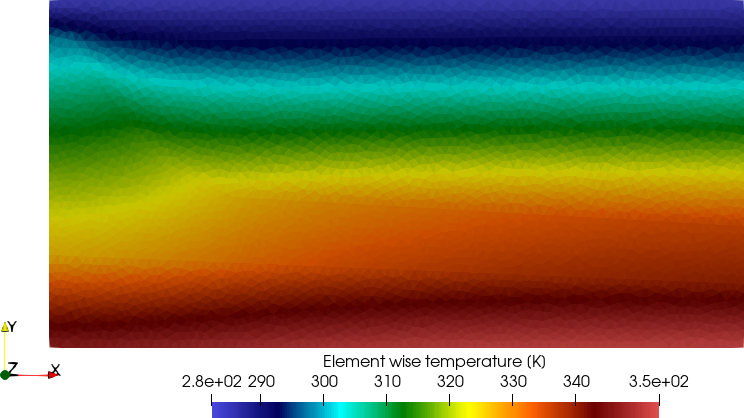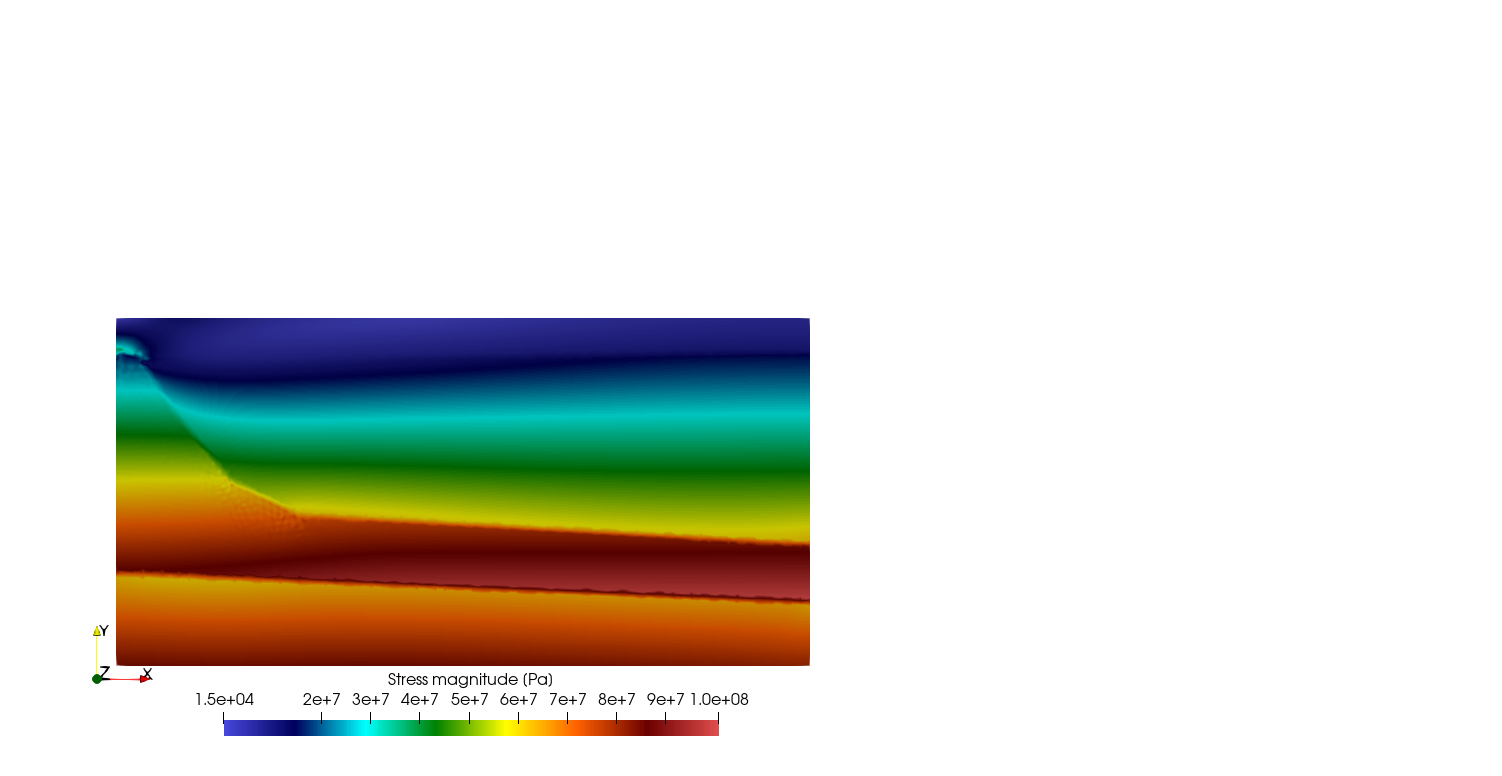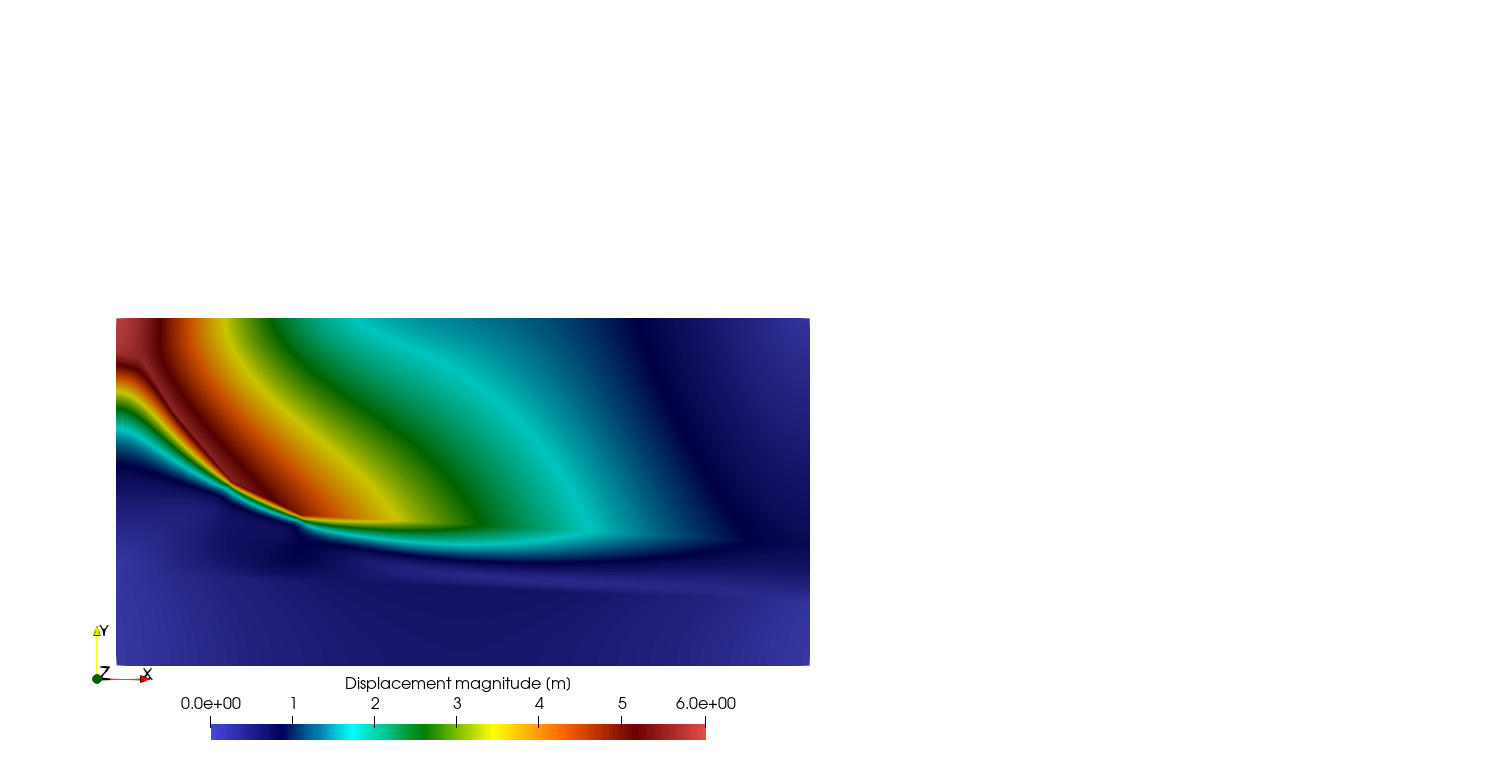# Creep analysis with a heterogeneous reference temperature

This plane strain creep problem is based on the conceptional model of salt dome presented in Bruns  and one of the benchmarks of the AREHS project (https://www.overleaf.com/project/5e908e022978e30001bc6b44). It is used here to test the use of the element wise distributed reference temperature.

The 2D domain is a rectangle with a size of 6000 m $\times$ 3000 m. The displacement in the normal direction of the lateral and the bottom boundaries are fixed. The top boundary is traction free. The BGRa creep model with the parameters of $A=0.18, \mbox{d}^{-1}$, $m=5$, $Q=54 \mbox{ kJ/mol}$ is used for the creep analysis. The other material parameters are listed in the following table:

Over burden Cap rock Salt rock Basement
Youngs’ modulus [GPa] 7.7 15.6 25.0 15.6
Poisson ratio 0.28 0.3 0.25 0.3
Density [kg/m$^3$] 1925.0 2700.0 2140.0 2700

The reference temperature is shown in the following figure:The initial stresses were obtained by conducting a simulation of the pure elastic deformation in the same domain under the gravitational force.

As a benchmark, only one thousand years’ creep with six time steps is considered.

The following two figures shown the results of stress magnitude and displacement magnitude at the last time step:Bruns, J. and Boetticher, L. and Doose, H. and Cottrell, M. and Wolff, P. and G{\"u}nther, R.M. and Naumann, D. and Popp, T. and Salzer, K. (2012): Glazigene Beeinflussung von Wirtsgesteinstypen Ton und Salz und deren Einflüsse auf die Eignung zur Aufnahme eines HAW-Endlagers. Abschlussbericht, vol. 292,